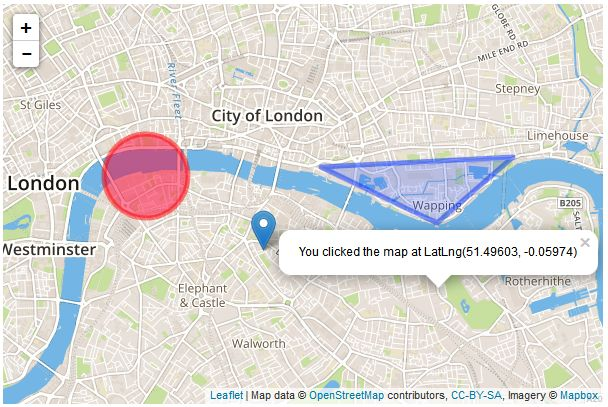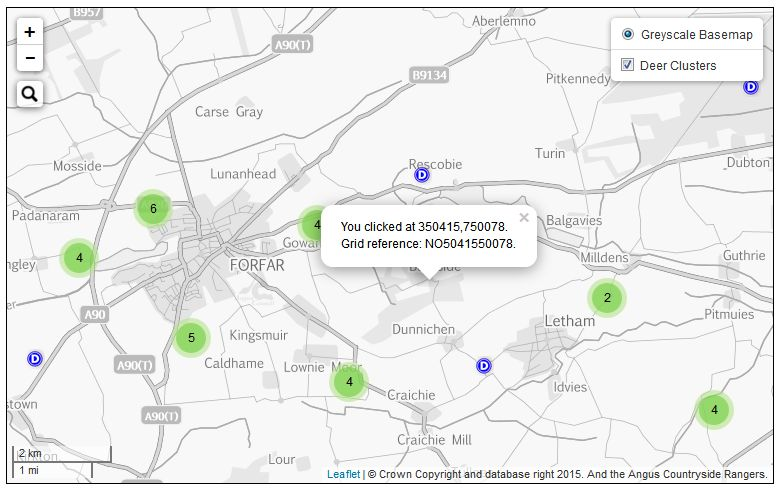# Reprojecting Leaflet Coordinates

Leaflet has a great little function that returns the coordinates of a point clicked on the map.

``````var popup = L.popup();

function onMapClick(e) {
popup
.setLatLng(e.latlng)
.setContent("You clicked the map at " + e.latlng.toString())
.openOn(map);
}

map.on('click', onMapClick);
``````

When the user clicks on the map the popup informs them of the coordinates of that point. In decimal degrees using the EPSG:4326 projection.What I wanted to do was get the coordinates in my projection - EPSG:27700 - and format the popup to present the information in a different way.After a quick search of gis.se I found this post which got me going in the right direction. To make this work correctly, converting the EPSG:4326 coordinates to EPSG:27700 coordinates, you need to add the following to the body of your HTML page:

``````<script type="text/javascript" src="https://cdnjs.cloudflare.com/ajax/libs/proj4js/2.3.10/proj4.js"></script>
``````

This adds the proj4 javascript library to help with reprojecting data and coordinates.

Then, in the body of your page edit the default Leaflet popup function to add a definition statement for the projection you want. In this case it is the British National Grid (BNG, EPSG:27700). Reproject the coordinates from longitude and latitude (degrees) to easting and northing (metres) using Proj4. There might be a better way to do this but I took the new coordinates in BNG, converted to a string, discarded everything past the decimal point to build 6-digit coordinates and then concatenated the same coordinates again to build a valid OS grid reference.

``````	// Create a popup to return clicked coordinates on the map
var popup = L.popup();
function onMapClick(e) {
var bngproj = '+proj=tmerc +lat_0=49 +lon_0=-2 +k=0.9996012717 +x_0=400000 +y_0=-100000 +ellps=airy +datum=OSGB36 +units=m +no_defs';
var bngcoords = proj4(bngproj, [e.latlng.lng, e.latlng.lat]);
popup
.setLatLng(e.latlng)
.setContent("You clicked at " + bngcoords.toString().substring(0,6) + bngcoords.toString().substring(17,25) + '<br/>' + "Grid reference: NO" + bngcoords.toString().substring(1,6) + bngcoords.toString().substring(19,25))
.openOn(map);
};
map.on('click', onMapClick);
``````